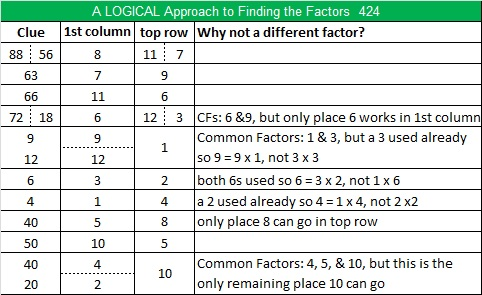# 424 and Level 5

The last two digits of 424 can be evenly divided by 4 so 424 can also be evenly divided by 4. Also because 24 is divisible by 8 and because the digit preceding the 24 is even, 424 is also divisible by 8. All of the factors of 424 are listed below the puzzle.Print the puzzles or type the factors on this excel file: 12 Factors 2015-03-09

• 424 is a composite number.
• Prime factorization: 424 = 2 x 2 x 2 x 53, which can be written 424 = (2^3) x 53
• The exponents in the prime factorization are 3 and 1. Adding one to each and multiplying we get (3 + 1)(1 + 1) = 4 x 2 = 8. Therefore 424 has exactly 8 factors.
• Factors of 424: 1, 2, 4, 8, 53, 106, 212, 424
• Factor pairs: 424 = 1 x 424, 2 x 212, 4 x 106, or 8 x 53
• Taking the factor pair with the largest square number factor, we get √424 = (√4)(√106) = 2√106 ≈ 20.5913This site uses Akismet to reduce spam. Learn how your comment data is processed.